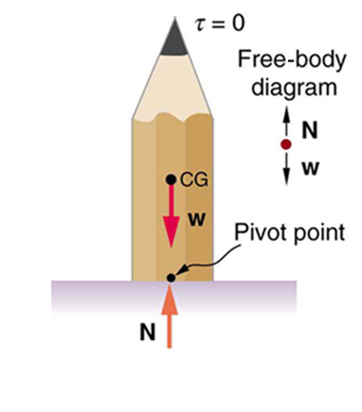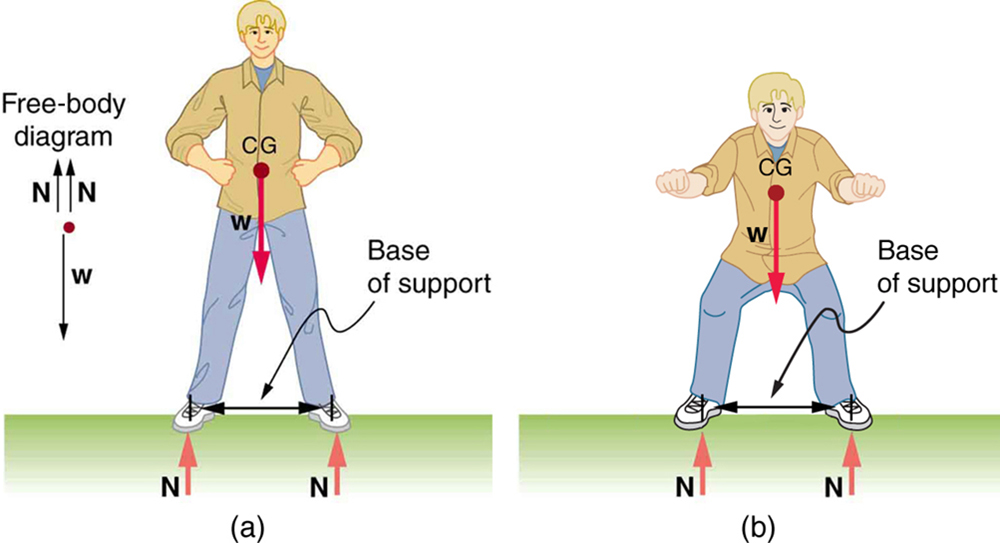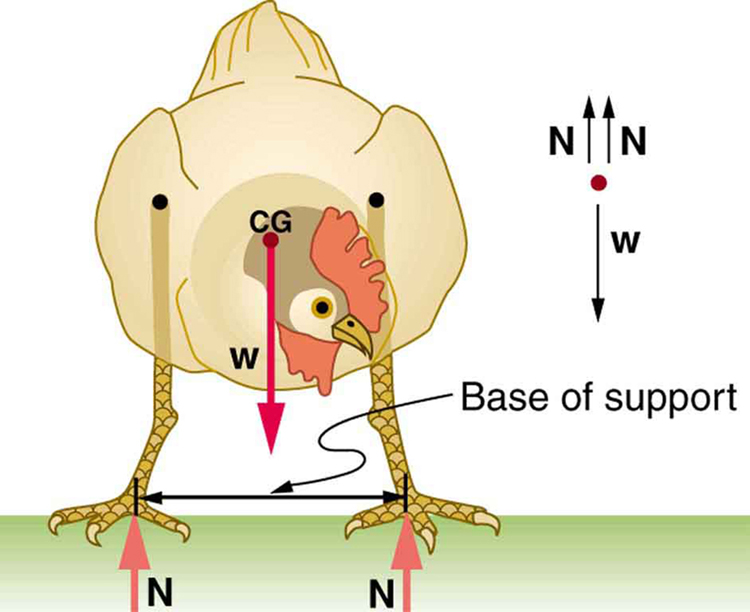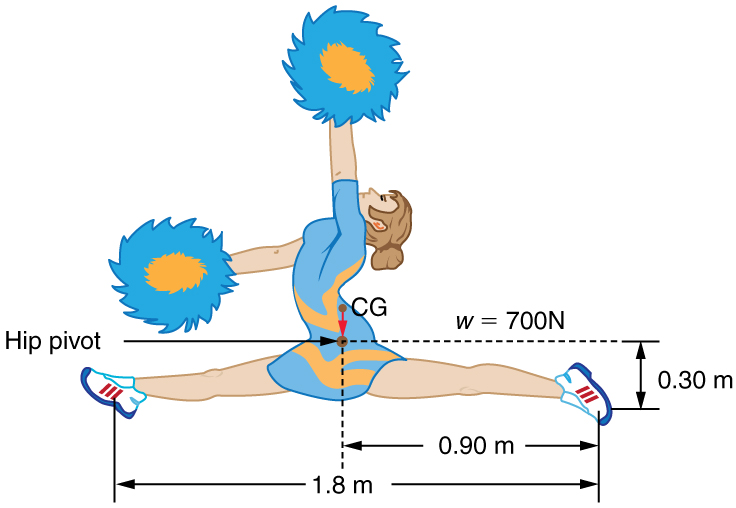# 60 8.3 Stability

### Summary

• State the types of equilibrium.
• Describe stable and unstable equilibriums.
• Describe neutral equilibrium.

It is one thing to have a system in equilibrium; it is quite another for it to be stable. The toy doll perched on the man’s hand in Figure 1, for example, is not in stable equilibrium. There are three types of equilibrium: stable, unstable, and neutral. Figures throughout this module illustrate various examples.

Figure 1 presents a balanced system, such as the toy doll on the man’s hand, which has its center of gravity (cg) directly over the pivot, so that the torque of the total weight is zero. This is equivalent to having the torques of the individual parts balanced about the pivot point, in this case the hand. The cgs of the arms, legs, head, and torso are labeled with smaller type.

A system is said to be in stable equilibrium if, when displaced from equilibrium, it experiences a net force or torque in a direction opposite to the direction of the displacement. For example, a marble at the bottom of a bowl will experience a restoring force when displaced from its equilibrium position. This force moves it back toward the equilibrium position. Most systems are in stable equilibrium, especially for small displacements. For another example of stable equilibrium, see the pencil in Figure 2.Figure 2. This pencil is in the condition of equilibrium. The net force on the pencil is zero and the total torque about any pivot is zero.

A system is in unstable equilibrium if, when displaced, it experiences a net force or torque in the same direction as the displacement from equilibrium. A system in unstable equilibrium accelerates away from its equilibrium position if displaced even slightly. An obvious example is a ball resting on top of a hill. Once displaced, it accelerates away from the crest. See the next several figures for examples of unstable equilibrium.

A system is in neutral equilibrium if its equilibrium is independent of displacements from its original position.

When we consider how far a system in stable equilibrium can be displaced before it becomes unstable, we find that some systems in stable equilibrium are more stable than others. The critical point is reached when the cg is no longer above the base of support. Additionally, since the cg of a person’s body is above the pivots in the hips, displacements must be quickly controlled. This control is a central nervous system function that is developed when we learn to hold our bodies erect as infants. For increased stability while standing, the feet should be spread apart, giving a larger base of support. Stability is also increased by lowering one’s center of gravity by bending the knees, as when a football player prepares to receive a ball or braces themselves for a tackle. A cane, a crutch, or a walker increases the stability of the user, even more as the base of support widens. Usually, the cg of a female is lower (closer to the ground) than a male. Young children have their center of gravity between their shoulders, which increases the challenge of learning to walk.Figure 7. (a) The center of gravity of an adult is above the hip joints (one of the main pivots in the body) and lies between two narrowly-separated feet. Like a pencil standing on its eraser, this person is in stable equilibrium in relation to sideways displacements, but relatively small displacements take his cg outside the base of support and make him unstable. Humans are less stable relative to forward and backward displacements because the feet are not very long. Muscles are used extensively to balance the body in the front-to-back direction. (b) While bending in the manner shown, stability is increased by lowering the center of gravity. Stability is also increased if the base is expanded by placing the feet farther apart.

Animals such as chickens have easier systems to control. Figure 8 shows that the cg of a chicken lies below its hip joints and between its widely separated and broad feet. Even relatively large displacements of the chicken’s cg are stable and result in restoring forces and torques that return the cg to its equilibrium position with little effort on the chicken’s part. Not all birds are like chickens, of course. Some birds, such as the flamingo, have balance systems that are almost as sophisticated as that of humans.

Figure 8 shows that the cg of a chicken is below the hip joints and lies above a broad base of support formed by widely-separated and large feet. Hence, the chicken is in very stable equilibrium, since a relatively large displacement is needed to render it unstable. The body of the chicken is supported from above by the hips and acts as a pendulum between the hips. Therefore, the chicken is stable for front-to-back displacements as well as for side-to-side displacements.Figure 8. The center of gravity of a chicken is below the hip joints. The chicken is in stable equilibrium. The body of the chicken is supported from above by the hips and acts as a pendulum between them.

The basic conditions for equilibrium are the same for all types of forces. The net external force must be zero, and the net torque must also be zero.

### TAKE-HOME EXPERIMENT

Stand straight with your heels, back, and head against a wall. Bend forward from your waist, keeping your heels and bottom against the wall, to touch your toes. Can you do this without toppling over? Explain why and what you need to do to be able to touch your toes without losing your balance. Is it easier for a woman to do this?

# Section Summary

• A system is said to be in stable equilibrium if, when displaced from equilibrium, it experiences a net force or torque in a direction opposite the direction of the displacement.
• A system is in unstable equilibrium if, when displaced from equilibrium, it experiences a net force or torque in the same direction as the displacement from equilibrium.
• A system is in neutral equilibrium if its equilibrium is independent of displacements from its original position.

### Problems & Exercises

1: Suppose a horse leans against a wall as in Figure 9. Calculate the force exerted on the wall assuming that force is horizontal while using the data in the schematic representation of the situation. Note that the force exerted on the wall is equal in magnitude and opposite in direction to the force exerted on the horse, keeping it in equilibrium. The total mass of the horse and rider is 500 kg. Take the data to be accurate to three digits.

2: Two children of mass 20.0 kg and 30.0 kg sit balanced on a seesaw with the pivot point located at the center of the seesaw. If the children are separated by a distance of 3.00 m, at what distance from the pivot point is the small child sitting in order to maintain the balance?

3: A person carries a plank of wood 2.00 m long with one hand pushing down on it at one end with a force F1 and the other hand holding it up at .500 m from the end of the plank with force F2. If the plank has a mass of 20.0 kg and its center of gravity is at the middle of the plank, what are the magnitudes of the forces F1 and F2?

4: A gymnast is attempting to perform splits. From the information given in Figure 10, calculate the magnitude and direction of the force exerted on each foot by the floor.Figure 10. A gymnast performs full split. The center of gravity and the various distances from it are shown.

## Glossary

neutral equilibrium
a state of equilibrium that is independent of a system’s displacements from its original position
stable equilibrium
a system, when displaced, experiences a net force or torque in a direction opposite to the direction of the displacement
unstable equilibrium
a system, when displaced, experiences a net force or torque in the same direction as the displacement from equilibrium

### Solutions

Problems & Exercises

1: $\boldsymbol{F_{\textbf{wall}}=1.43\times10^3\textbf{ N}}$

4: $\boldsymbol{350\textbf{ N}}$ directly upwards